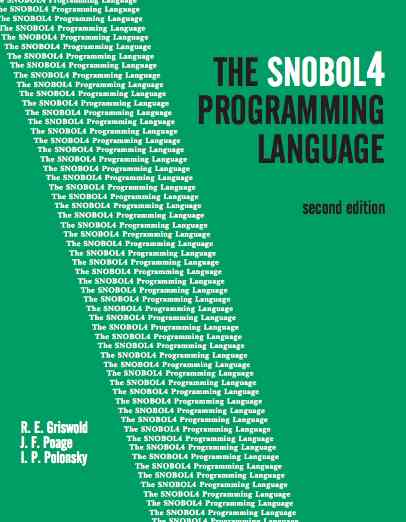#Shape RecognizerThe Snobol4 reference manual now available online from University of Arizona. pdfI can't remember what class had me writing Snobol but I'd already learned it on my own from a free short-course taught by Mike Shapiro.

Objects were to be identified as contiguous, two-dimensional spans of a single character. These were erased as found. The length of the last two lines determined the shape: increasing, decreasing or unchanged meant pyramid, ball, or block respectively.

This program read the following input that was provided by the instructor. Output was each shape, printed alone and appropriately classified.

```
11                        555
111                    5555555
11111                555555555
1111111             555555555
111111111           5555555
11111111111          555
1111111111111

99
9999999999999     9999
9999999999999     9999
9999999999999    999999
9999999999999    999999
99999999
99999999
9999999999

```

I can remember struggling with the logic until I put the pattern matcher in "full scan" which turned off search optimizations. This is a sure sign that I was abusing the matcher.

* PATTERN RECOGNITION PROGRAM * HOWARD CUNNINGHAM 11/15/72 FULLSCAN = 1; TRIM = 1 OUTPUT('PRINT',2,'(/1X,135A1)') LINE = ARRAY(20) * DEFINITIONS TOP.LINE = SPAN(' ') ?L (LEN(1) \$ C SPAN(*C)) . O ?R NEXT.LINE = ?L *LE(L,OR) SPAN(*C) . O ?R *GE(R,OL) * INPUT PICTURE INPUT OUTPUT = INPUT :F(TOP) LENGTH = LENGTH + 1 LINE<LENGTH> = OUTPUT :(INPUT) * LOCATE TOP OF OBJECT TOPN = 1; A = 0; PRINT = NEXT LINE<N> TOP.LINE = DUPL(' ',R) :S(SCAN) N = LT(N,LENGTH) N + 1 :S(NEXT) PRINT = 'ALL OBJECTS LOCATED' * SCAN TO BOTTOM OF OBJECT SCAN N = N + 1; A = A + R - L; K = OR - OL - R + L OR = R; OL = L; OUTPUT = DUPL(' ',L) O LINE<N> NEXT.LINE = DUPL(' ',R) :S(SCAN) * IDENTIFY KIND OF OBJECT L = 'PYRAMID'; L = EQ(K) 'BLOCK'; L = GT(K) 'BALL' PRINT = 'OBJECT IS ' L ' OF COLOR ' C ' AND SIZE ' + A ' CENTERED AT ' (OL + OR) / 2 :(TOP) END